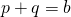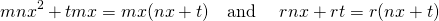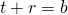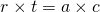# Factoring by decomposition proof

Suppose a trinomial factors with integers, such as:We can confirm the factors by multiplying out the brackets.

The method of decomposition states that given a factorable trinomial of the formwe can calculate the values in the brackets by finding two numbers that sum to the coefficientand that multiply to the product. On this page we explore why this method works.

We begin with the assumption that our trinomial does indeed factor. Let’s generalise:Let’s multiply out the right hand side:Our task is to figure out theand, given theandin the original trinomial.

Let’s compare the coefficients of the linewithWe notice thatSuppose we begin our search for factors by looking for the two numbers that add to the middle coefficient. As there are two unknowns, we need another piece of information to figure them out.

Suppose we call these two numbersand. Thenand.

When we multiplyandtogether, we getNotice that the product. That is, when we multiply these two values, our answer is the same asof the original trinomial.

We are ready to drop all the lettersand speak only ofand.

We need to find two numbersandsuch thatand.

We now have two conditions on our valuesandthat depend on the parametersandof the original trinomial.

# Why does the grouping always work out?

In our example, we haveAccording to our argument, we require to find two numbersandsuch thatandExamining the factors of 30 we have factor pairs (1,30); (2,15); (3,10); (5,6).

We see thatso we selectandor vice versa.

Now, when we decompose the middle term we haveorNo matter which way around we set theand the, grouping the first two terms and the last two terms will yield a common factor. Let’s see:or, vice versaEither way, we see a common factor emerge. Let’s examine the trinomial in terms ofagain:This line comes from the assumption that our trinomial can be factored.

Let’s factor the terms of the right hand side as we do for decomposition:we see thatis common to both terms.

Alternatively,here we see thatis common to both terms.

Either way, since our valuesandeach share one factor withand their other withthey can be exchanged.

# The simple caseNotice that in the simple case,. That is the linebecomesWe still require two numbers such thatandHowever, since in this case, the last line becomesOr, as we are used to saying ‘two numbers that add to the coefficient b and multiply to the coefficient c’.